+ +

# 10. Thick lens paraxial eye model

This page is devoted to the development of a paraxial thick lens pseudophakic eye model.

Once this model is established, we can explore the links that link the different biometric constants. This is an important step towards establishing a formula for calculating a thick lens paraxial IOL power calculation formula.

Classic paraxial biometric formulas were based on a thin lens eye model: the corneal power is estimated from the curvature of its anterior face, and the intraocular lens (IOL) is also likened to a thin lens. In this type of model, the axial length is equal to the distance between the cornea and the retinal photoreceptors. The position of the IOL is defined by its distance to the cornea.

Intraocular implants (IOLs) have a non-negligible thickness on the scale of the axial length of the human eye. In addition, the distribution of the curvatures between their anterior and posterior faces can vary according to the models and the powers, which implies that, as mentioned before, at an equal distance from the cornea, two implants of the same nominal power but different designs do not provide the same correction.

To overcome this problem, it is necessary to consider the thickness of the elements involved in ocular refraction: the cornea and the lens.

In thin lens models, it is assumed that the IOL’s anatomical position is equal to its « optical » position.  But what is most important to consider is that in a more realistic paraxial eye model using thick lenses, the anatomical position of the implant (defined by the distance between the vertex of the cornea and the vertex of the implant) is distinct from the « optical position » of the implant, which depends on the position of its principal planes.

The purpose of this page is to establish a relationship between the axial length of an emmetropic eye and the position of the IOL using a paraxial thick lens model.

It details the steps leading to the following relation:Where ALT and ELPT are, respectively, a modified axial length and an effective position of the modified IOL within a thick lens model framework.

This expression also depends on the value of the corneal power (Dc), the power of the implant (Di), and those of the refractive indices considered (na, nv). Why is it fundamental to establish such an equation? Because it offers the possibility of being resolved for any of the variables it contains, such as the ELPT (effective position of the IOL in a thick lens eye model), or Dc, the corneal power that provides emmetropia.

The following aims to justify this equation. You can ignore the derivation of this formula, but it would be a shame because it highlights many elements relating to the properties of models in thick lenses!

## Thick lens paraxial eye model: Gullstrand’s formula

Consider a thick-lens eye model consisting of a cornea and an implant. The cornea + implant system focuses incident light from a source located at infinity at a focal length F’e.

Gullstrand’s formula derives from paraxial approximations and makes it possible to integrate the effect of the thickness of a diopter or a lens (or the distance separating a pair of lenses) to calculate its optical power.

Using Gullstrand’s formula, we can show that De, the optical power resulting from the cornea + IOL pair, is equal to: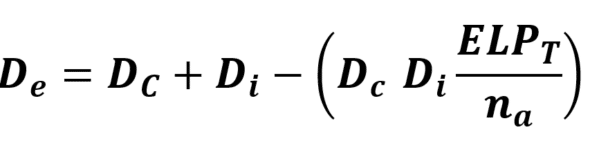ELPT is the optical distance between the cornea and the IOL. In a thick lens pseudophakic eye model, this distance corresponds to the distance between the principal image plane of the cornea and the principal object plane of the IOL.

The focal point of the optical system corresponding to the cornea + IOL (F’e) relative to the plane of the eye’s photoreceptors determines the refraction of the pseudophakic eye. The pseudophakic eye is emmetropic if the focal point F’e is located within this plane. If it is located in the front, the eye is myopic; if it is located behind, it is hyperopic.

## Thin vs. Thick lens eye paraxial eye model

For the same total powers of the cornea (Dc) and of the IOL (Di), for a thin lens eye model to be equivalent (to predict the same position for the focus F’e), it is necessary that the plane of the (thin) cornea coincide with the principal plane image of the (thick) cornea, and that the plane of the (thin)  IOL coincides with the main object plane of the (thick) IOL.

If this condition is met, then the thin lens model is equivalent (same position of the image focus of the couple cornea + IOL) to the thick lens model. The following figure is a schematic representation of this condition:

Using modern ocular optical biometry, anatomical data (axial length, corneal curvatures, thickness, anatomical depth of the anterior chamber, etc.) are acquired in clinical practice. Let’s take a look at how this data fits into a thick eye model.

## Effective lens position in a thick lens eye model (ELPT)

Let’s look at the thick pseudophakic eye model represented in the following figure. Some important dimensions appear, including the formula that links the ELPT to the anatomical position of the implant (S1S3 = ALP). Recall that the distances are algebraic (their positive or negative sign depends on whether the segments’ ends are stated from left to right or right under the upper line).

ALP is the anatomic lens position, from the anterior corneal vertex to the anterior IOL vertex

ILP is the internal lens position, from the posterior corneal vertex to the anterior IOL vertex.

One can write the relations between the ELPT and ALP as: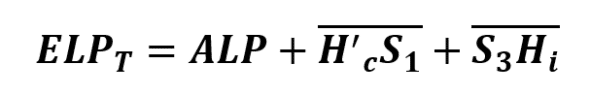Interestingly, H’cS1 and S3Hi depend only on the cornea and the IOL to be inserted, respectively. Therefore, they can be calculated if the information is available on the corneal topography and the type of IOL.

Only the ALP cannot be known in advance with certainty but it can be predicted. This is the main issue of IOL power calculation formulas.

We will now establish an expression that contains the main anatomical elements that play a role in calculating the power of the IOL. It is, therefore, necessary to establish relationships between the segments that, placed end to end, make up the anatomical axial length of an emmetropic pseudophakic eye.

## Anatomical vs. axial length in a thick lens paraxial eye model

The anatomical axial length (ALA) here corresponds to the value which connects the anterior corneal vertex to the photoreceptors’ plane at the fovea. Optical biometers provide the distance between the anterior corneal vertex and the retinal pigment epithelium using the technique of partial coherence interferometry.
In the case of an emmetropic eye, it is equal to the distance S1F’e, where F’e is the back focal point of the paraxial schematic pseudophakic eye.

We can split the anatomical axial length into two algebraic segments: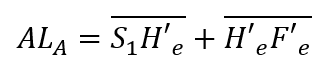H’eF’e is the image focal length distance of the pseudophakic eye, which is equal to the following ratio: nv/De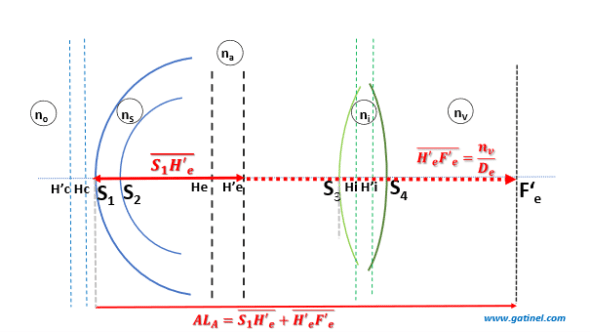S1H’c can be expanded using algebraic relations :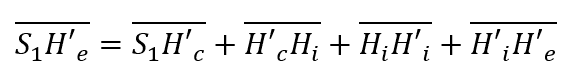H’cHi is the ELPT, and H’iH’e corresponds to the position of the principal image plane of the thick dual-lens paraxial eye model. The following expression gives it: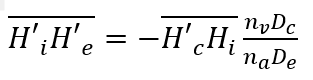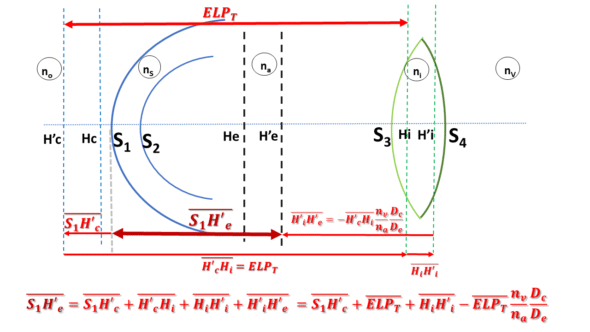Finally, after rearranging the terms in the preceding equations, we obtain the following: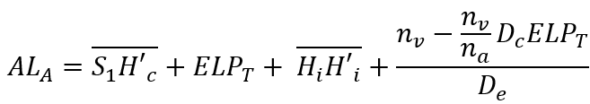Let us define :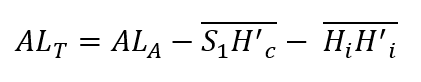ALT is equal to the anatomical axial length of the emmetropic eye reduced by the distance between the principal planes of the implant  HiH’i and the distance between the anterior surface of the cornea and the secondary principal plane of the cornea S1H’c (which is negative). The calculation of the ALT value can be carried out from data specific to the cornea of ​​the eye to be operated on and from the characteristics of the intraocular lens, which will have to be placed to replace the lens.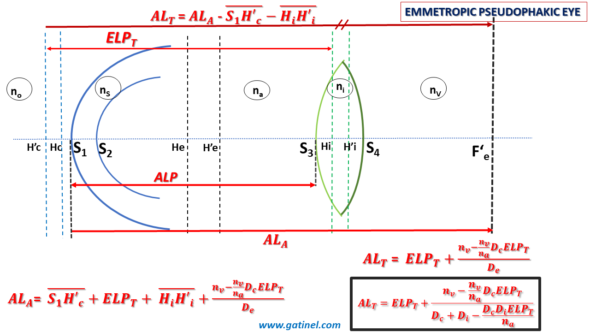We finally obtain the expression for an emmetropic pseudophakic eye:## Applications:

This equation is valuable:

It provides the value of the axial length of an emmetropic eye with a cornea and IOL-considered thick lenses.

One can resolve it for any of the variables it contains. This makes it possible to determine the value of certain variables in the circumstances such as:

calculating the value of the ELPT from the postoperative data. In this case, we must not lose sight of the fact that this expression concerns an emmetropic eye. In the case of a non-emmetropic eye, it is possible to modify the total vergence of the cornea to reduce in the case of an emmetropic eye.

Determining the total corneal power (Dc) is important in calculating the theoretical refraction (SE: spherical equivalent). Once this value is obtained, the refraction in the corneal plane is obtained by subtracting this value from real power measured using keratometry.

-last but not least, if we solve this equation for the power of the implant Di, we naturally obtain a biometric formula for calculating the power of IOL in a thick lens, paraxial eye model.

### 4 réponses à “10. Thick lens paraxial eye model”

1.JC Kim dit :

Dear Vincenzo,

Thank you so much for your help! I could finally make myself understood the thick lens principle!

2.Vincenzo Garomba dit :

Dear JC Kim. In this paper a thoroughly deduction of various parameters of interest is done:
https://doi.org/10.5897/IJPS2021.4950

3.Dr Damien Gatinel dit :

Thank you for your interest and kind comments. I am happy that you enjoy the website. Sorry for this insufficiently explained equation. It corresponds to the position of the paraxial image plane of the entire eye and is reported on this page (paraxial power of the pseudophakic eye) https://www.gatinel.com/14884-2/ (see the inserted figure). See also the plane dedicated to principal planes: https://www.gatinel.com/principal-planes-explanations/
I hope this helps!

4.JC Kim dit :

Thank you for your elaborate work. I am a big fan of this website from Korea.
However, there is a part difficult for me to understand.